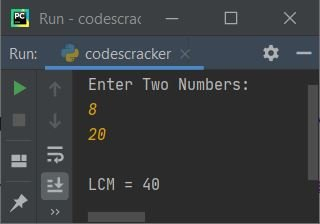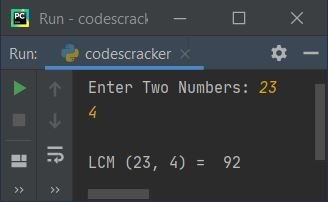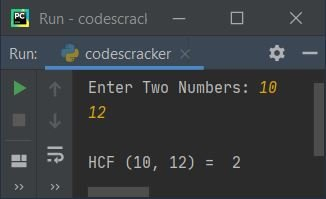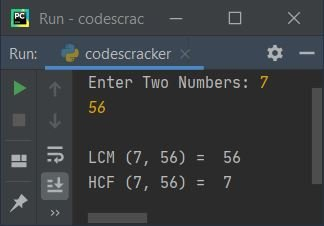# Python Program to Find LCM and HCF (GCD) of Two Numbers

This article is created to cover some programs in Python, that find and prints LCM and HCF (GCD) of two numbers entered by user. Here are the list of programs:

• Find LCM of Two Numbers
• Find HCF (GCD) of Two Numbers
• Find LCM and HCF of Two Numbers using Formula

### How to Find LCM/HCF ?

If you don't know the thing used to find LCM or HCF (GCD), then you can refer to any of the following, whatever you required, to get everything about the topic:

Note - LCM stands for Least Common Multiple. Whereas HCF stands for Highest Common Factor.

## Find LCM of Two Numbers

To find LCM of two numbers in Python, you have to ask from user to enter any two numbers, then find and print the LCM value as shown in the program given below. The question is, write a Python program to find LCM of two numbers. Here is its answer:

```print("Enter Two Numbers: ")
numOne = int(input())
numTwo = int(input())

if numOne>numTwo:
lcm = numOne
else:
lcm = numTwo

while True:
if lcm%numOne==0 and lcm%numTwo==0:
break
else:
lcm = lcm + 1

print("\nLCM =", lcm)```

Here is the initial output produced by this Python program:Now supply inputs say 8 as first number, then press `ENTER` key, again enter a number say 20 as second number, now press `ENTER` key to find and print LCM value of these two numbers as shown in the snapshot given below:The dry run of above program with same user input as provided in sample run, goes like:

• Initial values, numOne=8 (entered by user), numTwo=20 (entered by user)
• Now the condition (of if) numOne>numTwo or 8>20 evaluates to be true, therefore program flow goes inside this if's body and the value of numOne (8) gets initialized to lcm. So lcm=8
• If the condition was evaluated to be false, then program flow goes to else's body, instead of if's body
• Now the condition (of while loop) True always evaluates to be true, therefore program flow goes inside the loop
• And the first condition of if, that is lcm%numOne==0 or 8%8==0 evaluates to be true, therefore the second condition also gets evaluated. So the condition lcm%numTwo==0 or 8%20==0 evaluates to be false, therefore program flow goes to else's body and the value of lcm gets incremented by 1. So lcm=9
• Since the condition of while loop always evaluates to be true, therefore this process continues, until both the condition of if evaluates to be true
• That is, when both the condition of if evaluates to be true, then program flow goes inside its body and using break, the execution of while loop gets ended
• And after exiting from the loop, I've printed the value of lcm as LCM value of given two numbers
• So when the value of lcm becomes equal to 40, then the condition lcm%numOne==0 or 40%8==0 or 0==0 evaluates to be true, and the second condition lcm%numTwo==0 or 40%20==0 or 0==0 also evaluates to be true, therefore program flow goes inside the if's body and using break keyword, program flow goes out of the while loop
• In this way, the LCM of two numbers gets calculated

#### Modified Version of Previous Program

This is the modified version of previous program. This program uses end to skip inserting an automatic newline using print(). The str() converts any type of value to a string type. The try-except is used to handle and print error message, when user enters non-integer values.

```print("Enter Two Numbers: ", end="")
try:
nOne = int(input())
try:
nTwo = int(input())
if nOne > nTwo:
lcm = nOne
else:
lcm = nTwo
while True:
if lcm % nOne == 0 and lcm % nTwo == 0:
break
else:
lcm = lcm + 1
print("\nLCM (" + str(nOne) + ", " + str(nTwo)+") = ", lcm)
except ValueError:
print("\nInvalid Input!")
except ValueError:
print("\nInvalid Input!")```

Here is its sample run with user input, 23 and 4 as two numbers:## Find HCF (GCD) of Two Numbers

This program is created to find and print the value of HCF or GCD of two numbers entered by user at run-time:

```print("Enter Two Numbers: ", end="")
no = int(input())
nt = int(input())

if no>nt:
hcf = no
else:
hcf = nt

while True:
if no%hcf==0 and nt%hcf==0:
break
else:
hcf = hcf - 1

print("\nHCF (" + str(no) + ", " + str(nt) + ") = ", hcf)```

Here is its sample run with 10 and 12 as two numbers entered by user:## Find LCM and HCF using Formula

This program uses formula to find and print LCM and HCF both in a single program:

```print("Enter Two Numbers: ", end="")
no = int(input())
nt = int(input())

a = no
b = nt
while b!=0:
temp = b
b = a%b
a = temp

hcf = a
lcm = int((no*nt)/hcf)

print("\nLCM (" + str(no) + ", " + str(nt) + ") = ", lcm)
print("HCF (" + str(no) + ", " + str(nt) + ") = ", hcf)```

Here is its sample run with two numbers input as 7 and 56:#### Same Program in Other Languages

Python Online Test

« Previous Program Next Program »